Friday 10th July 2020

CBSE Guess > Papers > Question Papers > Class XII > 2004 > Physics > Outside Delhi Set-II

PHYSICS (Set II—Outside Delhi)

Except for the following questions, all the remaining questions have been asked in Set I.

Q. 4. Two metals A and B have work functions 4 eV and 10eV respectively. Which metal has higher threshold wavelength? 1

Q. 8. Draw a ray diagram, of an astronomical telescope in the near point adjustment. Write down the expression for its magnifying power. 2

Q. 9. Give the logic symbol for an AND gate. Draw the output wave form for AND gate for input wave forms A and B. 2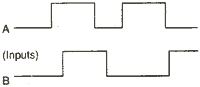Q. 10. Two long parallel straight wires X and Y separated by a distance of 2.5 cm in air carry currents of 5 A and 2.5 A respectively in opposite directions. Calculate the magnitude and direction of the force on a 10cm length of the wire Y. 2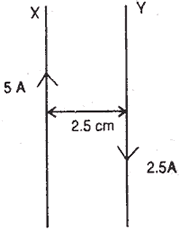Or

A circular coil of 200 turns, radius 5cm carries a current of 2.5 A. It is suspended vertically in a uniform horizontal magnetic field of 0.25 T, with the plane of the coil making an angle of 600 with the field lines.
Calculate the magnitude of the torque that must be applied on it to prevent it from turning.

Q. 11. Explain, with the help of graph, the variation of conductivity with temperature for a metallic conductor. 2

Q. 12. An electric dipole of length 8 cm, when placed with its axis making an angle of 600 with a uniform electric field experiences a torque of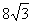Nm. Calculate the (i) magnitude of the electric field, (ii) potential energy of the dipole, if the dipole has charges of ± 4 nC. 2

Q. 14. Using Ampere’s circuital law, derive an expression for the magnetic field along the axis of a toroidal solenoid. 3

Or

A particle of mass ‘m’, with charge ‘q’ moving with a uniform speed ‘v’, normal to a uniform magnetic field ‘B’ describes a circular path of radius ‘r’. Derive expressions for the (i) time period of revolution and (ii) kinetic energy of the particle.

Q. 17. Calculate the binding energy per nucleon of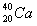nucleus
[Given: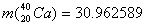mn (mass of a neutron) =1.008665 u
mp (mass of a proton) =1.007825 u]

Q. 21. Explain briefly the principal of transmitting signals using a satellite. State two main advantages of using a satellite for transmitting signals. 3

Q. 24. A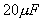capacitor is charged by a 30 V d. C. supply and then connected across an uncharged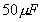capacitor. Calculate (i) the final potential difference across the combination, and (ii) the initial and final energies. How will you account for the difference in energy? 3

 Physics 2004 Question Papers Class XII Delhi Outside Delhi Compartment Delhi Compartment Outside DelhiSet ISet ISet ISet ISet IISet IISet IISet IISet IIISet III

CBSE 2004 Question Papers Class XII